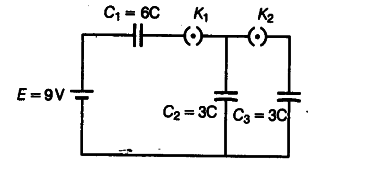# In the circuit shown in figure

In the circuit shown in figure, initially { K }_{ 1 } is closed and
{ K }_{ 2 } is opened.
What are the charges on each of the capacitors? Then,{ K }_{ 1 } was opened and
{ K }_{ 2 } was closed (order is important), what will be the charge on each capacitor now?In the circuit, when initially { K}_{1 } is closed
and { K}_{2} is opened, the capacitors
{ C}_{1 } and
{ C}_{2} acquire potential difference
{ V}_{1 } and
{ V}_{2 }, respectively.
So we have